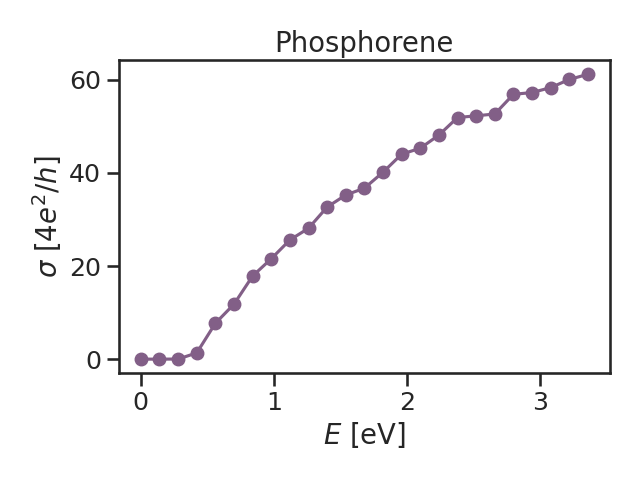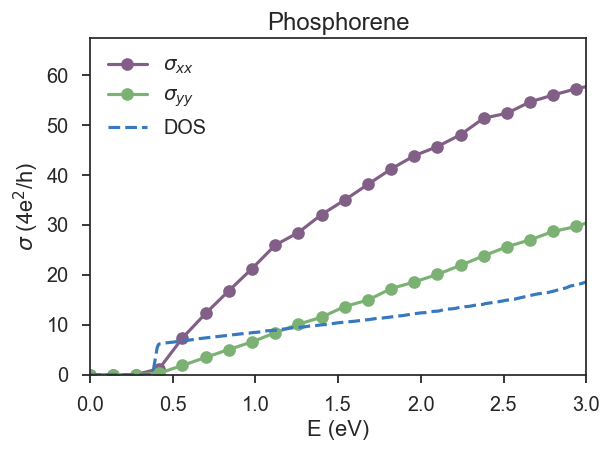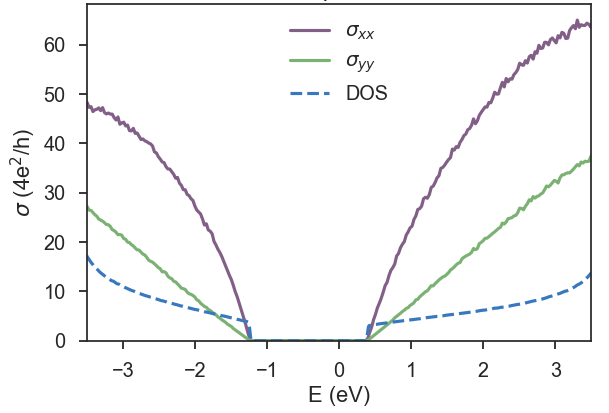# Phosphorene

### Phosphorene¶

This is a small tutorial to illustrate the use of KITE to investigate materials with anisotropic electrical conductivity. To this end, we consider a simplified tight-binding model for single layer phosphorene[^1]. Even though this model is very simple, it captures the anisotropic band structure of phosphorene, which is Dirac like in one direction and Schrödinger like in the other direction. This behaviour results in highly anisotropic transport properties along the different directions[^2].

We calculate the single energy longitudinal conductivity (singleshot_conductivity_dc) in the vicinity of the band gap. This is a fast numerical calculation, that is set to run in a normal laptop for about 3-4 minutes. It can reproduce qualitatively the expected anisotropic conductivity along xx and yy directions.

Here, we highlight parts of the python scripts. The complete scripts can be downloaded for the xx conductivity and for the yy conductivity.

#### Lattice¶

After the imports that are necessary for KITE, we define the lattice, with Pybinding:

  1 2 3 4 5 6 7 8 9 10 11 12 13 14 15 16 17 18 19 20 21 22 23 24 25 26 27 28 29 30 31 32 33 34 35 36 37 38 39 40 41 42 43 44 45 46 47 48 49 50 51 52 53 54 55 56 57 58 59 60 from numpy import cos, sin, pi def monolayer_4band(num_hoppings=4): """Monolayer phosphorene lattice using the four-band model Parameters ---------- num_hoppings : int Number of hopping terms to consider: from t2 to t5. """ a = 0.222 # nm ax = 0.438 # nm ay = 0.332 # nm theta = 96.79 * (pi / 180) phi = 103.69 * (pi / 180) lat = pb.Lattice(a1=[ax, 0], a2=[0, ay]) h = a * sin(phi - pi / 2) s = 0.5 * ax - a * cos(theta / 2) lat.add_sublattices(('A', [-s/2, -ay/2, h], 0), ('B', [ s/2, -ay/2, 0], 0), ('C', [-s/2 + ax/2, 0, 0], 0), ('D', [ s/2 + ax/2, 0, h], 0)) lat.register_hopping_energies({'t1': -1.22, 't2': 3.665, 't3': -0.205, 't4': -0.105, 't5': -0.055}) if num_hoppings < 2: raise RuntimeError("t1 and t2 must be included") elif num_hoppings > 5: raise RuntimeError("t5 is the last one") if num_hoppings >= 2: lat.add_hoppings(([-1, 0], 'A', 'D', 't1'), ([-1, -1], 'A', 'D', 't1'), ([ 0, 0], 'B', 'C', 't1'), ([ 0, -1], 'B', 'C', 't1')) lat.add_hoppings(([ 0, 0], 'A', 'B', 't2'), ([ 0, 0], 'C', 'D', 't2')) if num_hoppings >= 3: lat.add_hoppings(([ 0, 0], 'A', 'D', 't3'), ([ 0, -1], 'A', 'D', 't3'), ([ 1, 1], 'C', 'B', 't3'), ([ 1, 0], 'C', 'B', 't3')) if num_hoppings >= 4: lat.add_hoppings(([ 0, 0], 'A', 'C', 't4'), ([ 0, -1], 'A', 'C', 't4'), ([-1, 0], 'A', 'C', 't4'), ([-1, -1], 'A', 'C', 't4'), ([ 0, 0], 'B', 'D', 't4'), ([ 0, -1], 'B', 'D', 't4'), ([-1, 0], 'B', 'D', 't4'), ([-1, -1], 'B', 'D', 't4')) if num_hoppings >= 5: lat.add_hoppings(([-1, 0], 'A', 'B', 't5'), ([-1, 0], 'C', 'D', 't5')) lat.min_neighbors = 2 return lat 

This model, as defined above, can be used with different number of hoppings. The user can decide the number that is used in the calculation when defining the lattice:

lattice=monolayer_4band(num_hoppings=4)


### KITE-part¶

#### Settings¶

To use the large-scale single-shot algorithm for direct evaluation of zero-temperature DC conductivities, the resolvent operator requires a nonzero broadening (resolution) parameter eta, which is given in eV. As this type of calculation is energy dependent, it is also necessary to provide a list of desired energy points to the calculation object. In the single shot calculations, the computational time scales linearly with the energy points. For this example, that is intended to run in a normal desktop, we consider a small number of points and the energy range is set in the vicinity of the band gap.

The number of points and the list of energy points can be created when calling the calculation, as illustrated here:

 1 2 3 4 5 6 7 8 9 calculation = kite.Calculation(configuration) npoints = 25 epoints = [(1.0 / npoints * i) * 3.5 for i in range(npoints)] calculation.singleshot_conductivity_dc(epoints, num_moments=512, num_random=5, num_disorder=1, direction='xx', eta=0.02) 

Now it is time to save the configuration in a hdf file:

    kite.config_system(lattice, configuration, calculation, filename='phxx.h5')


#### Visualization¶

After running KITEx no post-processing is required for the singleshot conductivity calculation. The result can be extracted and plotted with the following script:

  1 2 3 4 5 6 7 8 9 10 11 12 13 14 15 16 17 18 19 20 21 22 23 24 25 26 27 28 29 30 31 import h5py import matplotlib.pyplot as plt import matplotlib as mpl import seaborn as sns mpl.rcParams['figure.dpi'] = 100 mpl.rcParams['savefig.dpi'] = 100 sns.set_style("white") # Kite color scheme colors = ["dusty purple", "faded green", "windows blue", "amber", "greyish"] current_palette = sns.xkcd_palette(colors) sns.set_palette(current_palette) sns.set_style("ticks") sns.set_context("talk", font_scale=1.1) file_name = 'phxx.h5' num_points = 25 energy = [(1.0 / num_points * i) * 3.5 for i in range(num_points)] file_input = h5py.File(file_name, 'r+') single_shot = file_input['Calculation']['singleshot_conductivity_dc']['SingleShot'] cond = single_shot[:, 3] plt.plot(energy, cond, 'o-') plt.xlabel('$E$ [eV]') plt.ylabel(r'$\sigma$ [$4e^2 / h$]') plt.title('Phosphorene') plt.tight_layout() plt.show()It is not possible to request the same type of calculation in a single call. In this case, we want to calculate the conductivity in xx and yy directions where the type of the calculation is the same, which means we need another hdf file for yy conductivity.

Let's repeat the procedure for another direction:

 1 2 3 4 5 6 7  calculation.singleshot_conductivity_dc(epoints, num_moments=512, num_random=5, num_disorder=1, direction='xx', eta=0.02) kite.config_system(lattice, configuration, calculation, filename='phyy.h5') 

The result of this fast calculation can be seen in the figure below, for l1=512, l2=512. To get a feeling of how KITE works, we suggest modifying parameters like eta and num_random.In the next figure, we repeat the calculation for 300 energy points and 10 random vectors and a large energy window.[^1] Alexander N. Rudenko, Mikhail I. Katsnelson, Phys. Rev. B 89, 201408 (2014).

[^2] H. Liu, A. T. Neal, Z. Zhu, X. Xu , D. Tomanek and P. D. Ye, ACS Nano 8, 4033 (2014)